Data Representation Model Notation
 ` ` The SEDRIS DRM is based on Rational's Unified Modeling Language (UML) notation. The SEDRIS DRM uses some minor extensions (i.e., extra notations) to the UML but, for the most part, the notation is UML. The UML notation follows the object-oriented methodology for organizing data. Class Notation Central to the UML notation is the concept of a class of data. A class is an abstract, user-defined description of a type of data. It identifies the attributes of the data and the operations that can be performed on instances (i.e., objects) of the data. This modeling technique allows description of a system using the same terminology as the corresponding real-world objects and their associated characteristics. In SEDRIS, the data representation model contains the classes necessary for the representation of the natural environment, not abstractions of the objects in the natural environment. The SEDRIS DRM does not contain a class for a "tree" or a "tank", but instead contains the classes that are required to represent a tree or a tank, or any other kind of object found in the natural environment. A class of data has a name, a set of attributes that describe its characteristics, and a set of operations that can be performed on the objects of that class. (NOTE: A "method" is an implementation of an operation.) In UML notation, a class is represented by a rectangular box having three segments.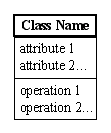To minimize the amount of information on the SEDRIS DRM diagrams, all the attribute information is placed in the data dictionary. Since the data defined by the SEDRIS DRM are used to capture the contents of an environmental database, they do not experience dynamic changes. Therefore, no operations are defined for any of the SEDRIS classes of data. In the SEDRIS DRM diagrams, a class is represented by a single rectangle with the Class Name.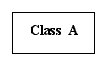The SEDRIS DRM contains two kinds of classes - abstract and concrete. Abstract classes are never instantiated (i.e., no objects of this class are created). Concrete classes can have objects created from them. Abstract classes are used to define the common attributes that may be used by any of its subordinate (i.e., child) classes - an inheritance relationship. In the SEDRIS DRM diagrams, abstract classes are denoted by a "shaded" rectangle with the Class Name in italics. (Shading rectangles to denote abstract classes is a deviation from the UML notation.)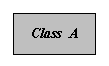Logical Relationships Critical items of information to be captured in the DRM diagrams are the logical relationships between the classes of data. There are three kinds of relationships between classes of data: association, inheritance, and aggregation. Each type of relationship is represented by a different notation. Association Relationship and Multiplicity A direct line between two classes denotes the weakest of relationships: association. The following notation indicates that every object in Class A is associated with exactly one object in Class B, and that every object in Class B is associated with exactly one object in Class A. (In SEDRIS, if one object is associated with another object, then the two objects are different representations of the same "real world" object.)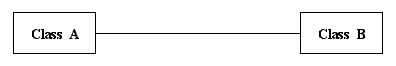Numerals are used at either end (or both ends) of the association relationship to convey multiplicity of each class. In the above notation, the numeral "1" means "exactly one". The "0..1" notation is used to denote "zero or one". The following notation indicates that every object in Class A is associated with zero or one object in Class B, and that every object in Class B is associated with exactly one object in Class A.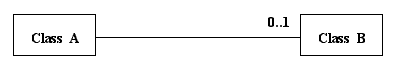The "0..*" notation is used to denote "zero or more" (many). The following notation indicates that every object in Class A is associated with zero or more objects in Class B, and that every object in Class B is associated with exactly one object in Class A. ("Zero or more" is alternately represented as merely "*" or no numeral at all, implying undefined or many.)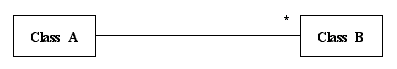A numeral followed by a "..*" is used to denote a specific "range" (i.e., N..* means "at least n or more"). The following notation indicates that every object in Class A is associated with "at least 3 or more" objects in Class B, and that every object in Class B is associated with exactly one object in Class A.An "{ordered}" annotation is used to denote that a collection of objects is "ordered". Otherwise, a collection of objects is assumed to have no particular order. The following notation indicates that the ordering of the objects in Class B is important to the relationship shared between Class A and Class B.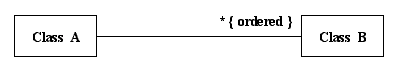An "open arrow" is used to denote a "one-way" association. The following notation indicates that every object in Class A is associated with zero or more objects in Class B, and that every object in Class B is associated with exactly one object in Class A. However more importantly, this notation indicates that a Class A object will "know" what Class B objects with which it is associated, and that a Class B object will "not know" what Class A object with which it is associated.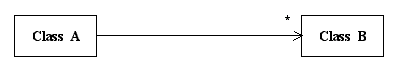A "dashed line connected to the association relationship line" is used to denote an "association" or "link" class, indicating that the association relationship between two classes has its own attributes. The following notation indicates that every object in Class A is associated with zero or more objects in Class B, and that every object in Class B is associated with exactly one object in Class A. It also indicates that each (Class A, Class B) object pair is associated with exactly one object in Class C.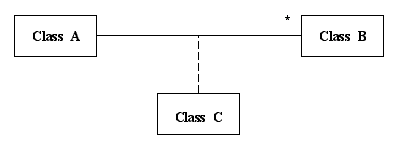Generalization Relationship A "solid line with a large hollow triangle" used to connect lines between two classes denotes the generalization (or inheritance) relationship. The following notation indicates that Class A is the Parent or Superclass, while Classes B and C are the Child or Subclasses. Objects in Classes B and C "inherit" the attributes of Class A (their parent), while having additional unique attributes of their own. This relationship is sometimes referred to as the "is-a" relationship - an object in Class B or Class C "is-a" type of Class A. There are no multiplicity numerals used with the generalization relationship, since the SEDRIS DRM does not support multiple inheritance. The "is-a" relationship is always one-to-one. (Excluding the representation of multiple inheritance relationships is a deviation from the UML notation.)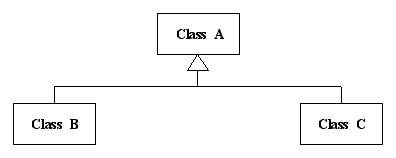Aggregation Relationship A "diamond" used to connect lines between two classes denotes the aggregation relationship. The following notation indicates that Class A is an aggregation of (or contains) objects of Class B. In other words, Class A "has-a" Class B. The aggregation relationship may also appear with multiplicity numerals, as described above.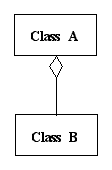An Illustrative Example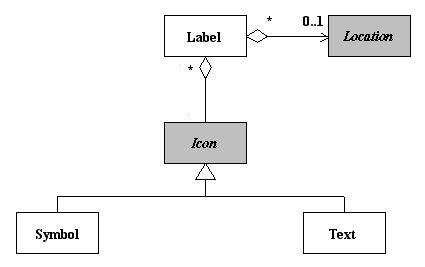The meaning of this notation is described below. - The
 Last updated: October 8, 2004